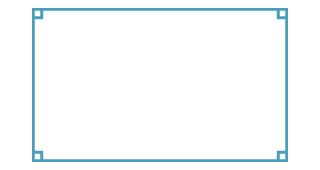## Rectangles

######## Definition of a rectangle

A rectangle is a quadrilateral in which all angles are right angles.

##### Properties of a rectangle
• A rectangle is a parallelogram, so its opposite sides are equal.
• The diagonals of a rectangle are equal and bisect each other.
##### Tests for a rectangle
• A parallelogram with one right angle is a rectangle.
• A quadrilateral whose diagonals are equal and bisect each other is a rectangle.